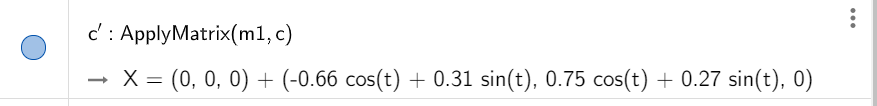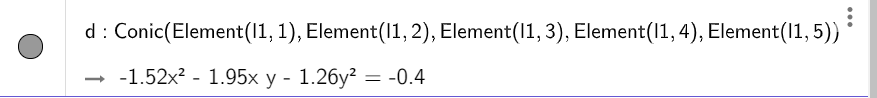# How to get the expression of the projection of a 3D circle on xOyPlane.

Nicholasfox shared this question 2 years ago

How to get the expression of the projection of a 3D circle on xOyPlane?

I've tried locusequation command but faild.I'm sure there's a nicer way, but this works

```Sequence((x(Point(c, i)), y(Point(c, i))), i, 0, 0.9, 0.2)
Conic(Element(l1, 1),Element(l1, 2),Element(l1, 3),Element(l1, 4),Element(l1, 5))```3

I'm sure there's a nicer way, but this works

```Sequence((x(Point(c, i)), y(Point(c, i))), i, 0, 0.9, 0.2)
Conic(Element(l1, 1),Element(l1, 2),Element(l1, 3),Element(l1, 4),Element(l1, 5))```1

Unbelievable! Thank you very much.3

I do not know if nicer way

m1 = {{1, 0, 0}, {0, 1, 0}, {0, 0, 0}}

ApplyMatrix(m1, c)1

Thanks.

Is there a easy way to transform Parametric equations into Conic equations.

from:to:1

only the michael method. create five points and use conic tool3

he hecho varios intentos pero siempre se produce un objeto en 3D y para tener una conica hay que reducirlo a 2D usando coordenadas x e y

Files: foro.ggb1

Have a lesson, thanks.